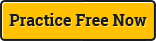Double-entry book keeping is a system of accounting. It entails each transaction being listed in at least two different ledger accounts. Each transaction is recorded as a debit in one account, and a credit in the other. The debits and credits must cancel one another out. Although the layperson considers crediting as an increase in something, whether or not a credit increases the value of the account is dependent upon the type of account. For instance, debiting an asset is an increase in the asset. The accounting equation (also known as the golden rule of accounting) need always be remembered. That is, equity=assets – liabilities. Put another way, assets=equity + liabilities. That is, the balance sheet must balance.

QUESTION:

If assets are equal to 100, then the sum of equity and liabilities must be 100 in order for the balance sheet to balance.

We recommend practicing these tests under real timed test conditions. Take a free timed verbal test right now.

EXPLANATION:

Penultimate sentence says assets=equity + liabilities and the last sentence says this will balance the balance sheet.Numerical reasoning testsDiagrammatic reasoning testsVerbal reasoning tests